## 2. Background

Using devices such as Jawbone Up, Nike FuelBand, and Fitbit it is now possible to collect a large amount of data about personal activity relatively inexpensively. These types of devices are part of the quantified self-movement. A group of enthusiasts who take measurements about themselves regularly to improve their health, to find patterns in their behavior, or because they are tech geeks. One thing that people regularly do is quantify how much of a activity they do, but they rarely quantify how well they do it. In this project, your goal will be to use data from accelerometers on the belt, forearm, arm, and dumbbell of 6 participants. They were asked to perform barbell lifts correctly and incorrectly in 5 different ways.

### a. Data Source

The training data and the testing data are below:

### b. Environment Setup

if(!require("pacman")) install.packages("pacman")
pacman::p_load(knitr, corrplot, caret, randomForest, rattle, gbm)
options(digits = 3)
set.seed(123)

# link set
train.file = "pml-training.csv"
test.file = "pml-testing.csv"

# partition dataset
in.train = createDataPartition(train$classe, p = 0.7, list = F) train.set = train[in.train, ] test.set = train[-in.train, ] # check dataset dim(train.set) ##  13737 160 Firstly, we are gonna clean the NA variables and identification variables. Importantly, the test dataset is not changed and will only be used for the quiz results generation. # near zero variance nzv = nearZeroVar(train.set) train.set = train.set[, -nzv] # check dataset dim(train.set) ##  13737 111 The variables as near zero variance are meaningless for modeling. # mostly NA variable near.na = sapply(train.set, function(x) mean(is.na(x))) > 0.95 train.set = train.set[, near.na == F] # check dataset dim(train.set) ##  13737 59 The variables as mostly NA variables are useless for modeling. # identification variable train.set = train.set[, -c(1:5)] # check dataset dim(train.set) ##  13737 54 The variable as identification variables are pointless for modeling. After cleaning, we can see the ready-to-analysis dataset has 53 variables as independent and 1 variable as dependent. ### d. Exploring Data Analysis cor.matrix = cor(train.set[, -54]) corrplot(cor.matrix, order = "FPC", method = "color", type = "lower", tl.cex = 0.6, tl.col = rgb(0, 0, 0))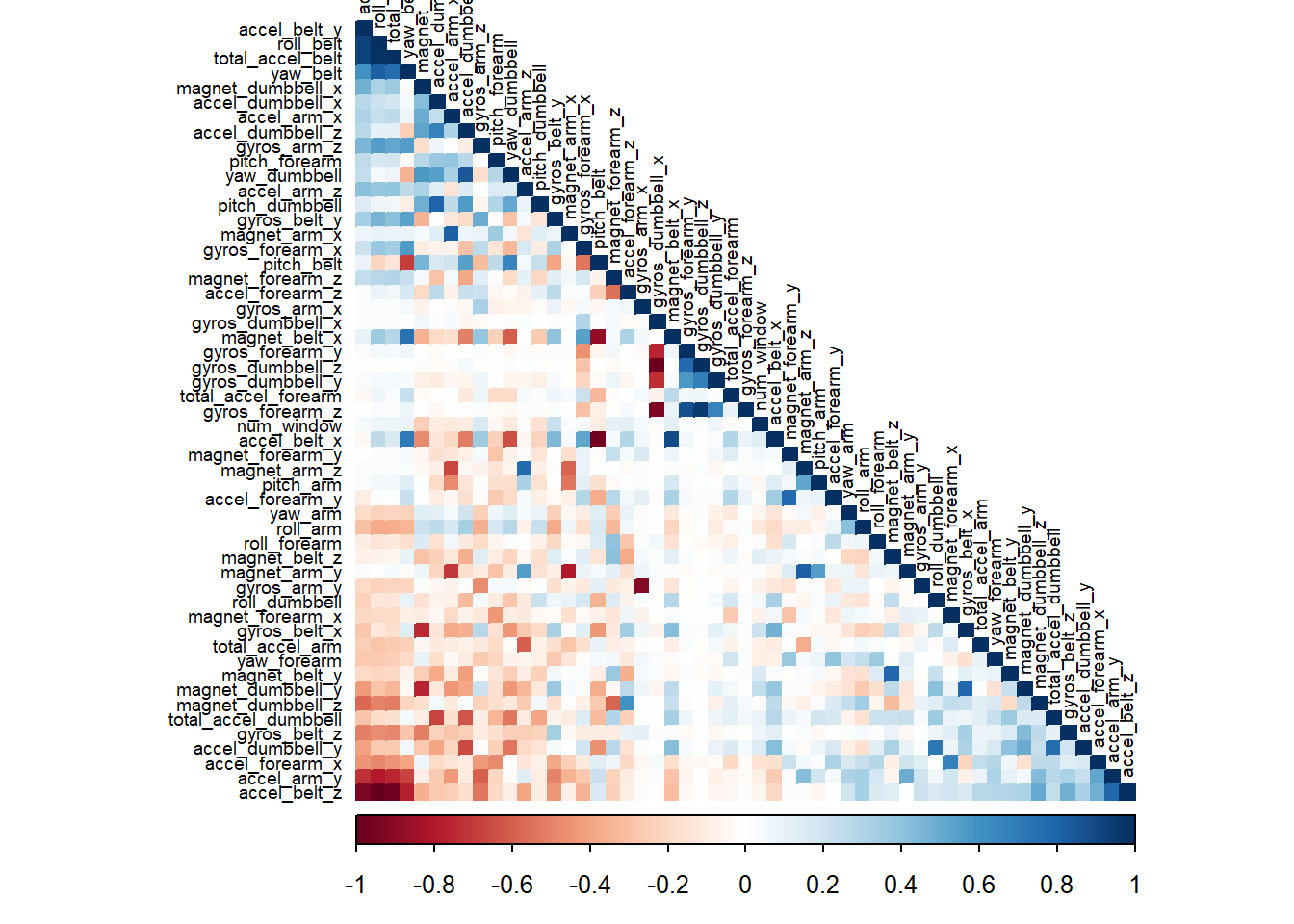A correlation among variables is analysed before modeling. If the correlations are quite more, a principal components analysis (PCA) could be performed as processing step to make an even more compact analysis. However, the plot shows quite a few correlations. PCA will not be applied. ## 4. Model Building We are gonna use three methods to build up models and even stack them together. The methods are random forest, decision tree, and generalized boosted model. ### a. Random Forest # train model mod.rf = train(data = train.set, classe ~ ., method = "rf", trControl = trainControl(method = "cv", number = 3)) # test model & validate model pred.rf = predict(mod.rf, newdata = test.set) # evaluate model cm.rf = confusionMatrix(table(pred.rf, test.set$classe))

# plot matrix result
plot(cm.rf$table, col = cm.rf$byClass,
main = "Random Forest",
sub = paste("Accuracy =", round(cm.rf$overall, 4)), xlab = "Prediction", ylab = "Reference")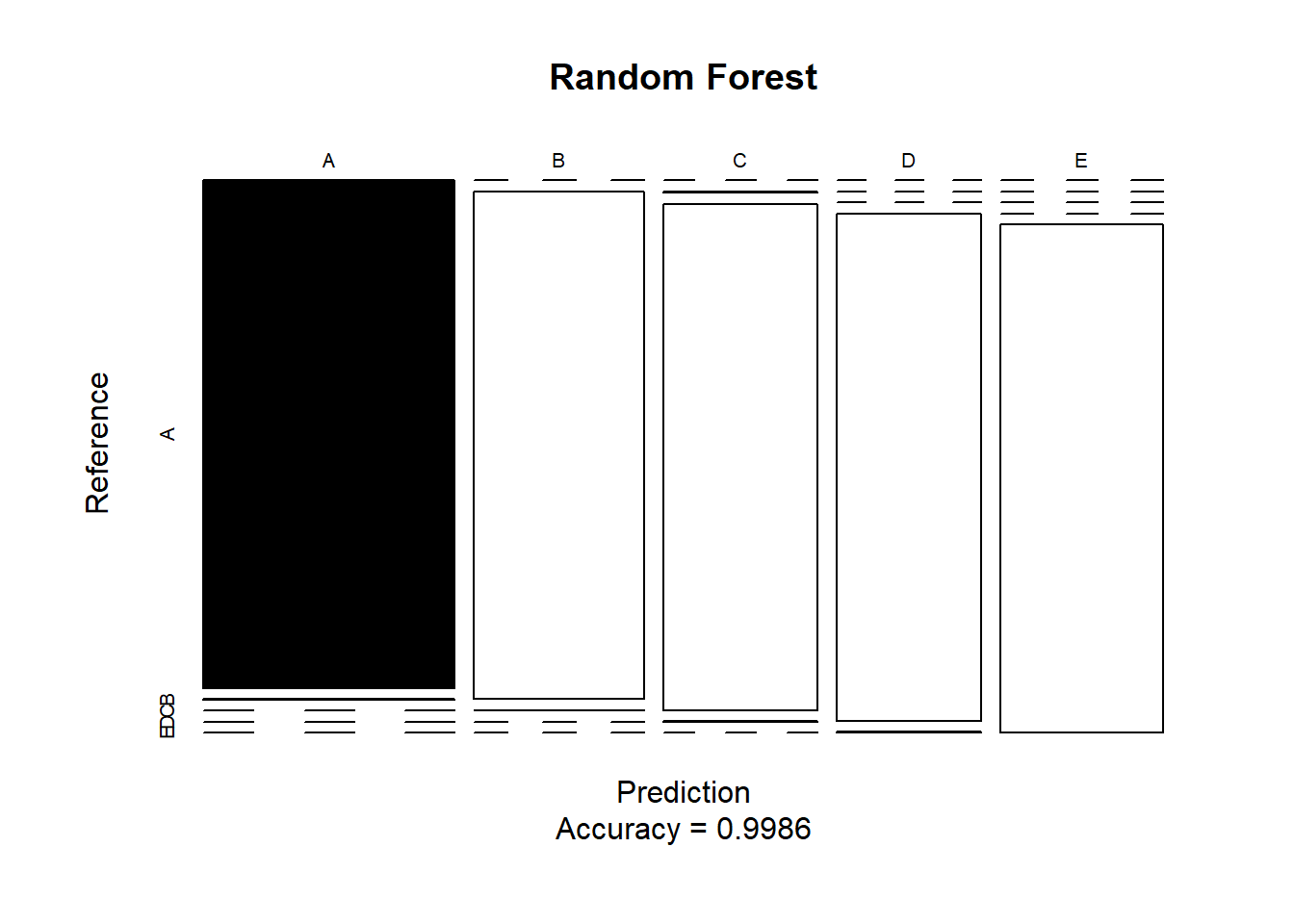### b. Decision Tree # train model mod.dt = train(data = train.set, classe ~ ., method = "rpart", trControl = trainControl(method = "cv", number = 3, verboseIter = F)) fancyRpartPlot(mod.dt$finalModel) # decision tree plot (optional)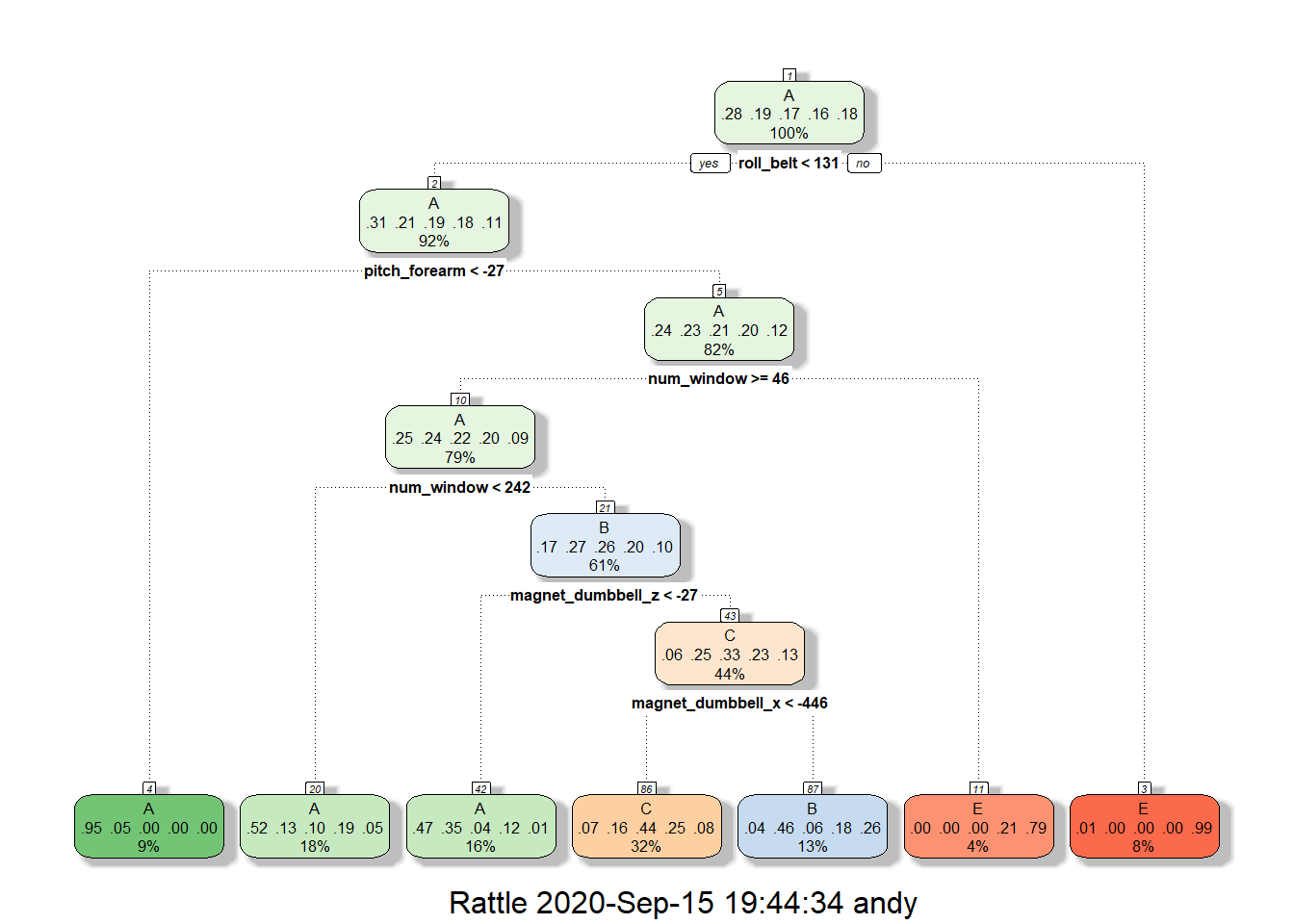# test model & validate model
pred.dt = predict(mod.dt, newdata = test.set)

# evaluate model on validate
cm.dt = confusionMatrix(table(pred.dt, test.set$classe)) # plot matrix result plot(cm.dt$table, col = cm.dt$byClass, main = "Decision Tree", sub = paste("Accuracy =", round(cm.dt$overall, 4)),
xlab = "Prediction",
ylab = "Reference")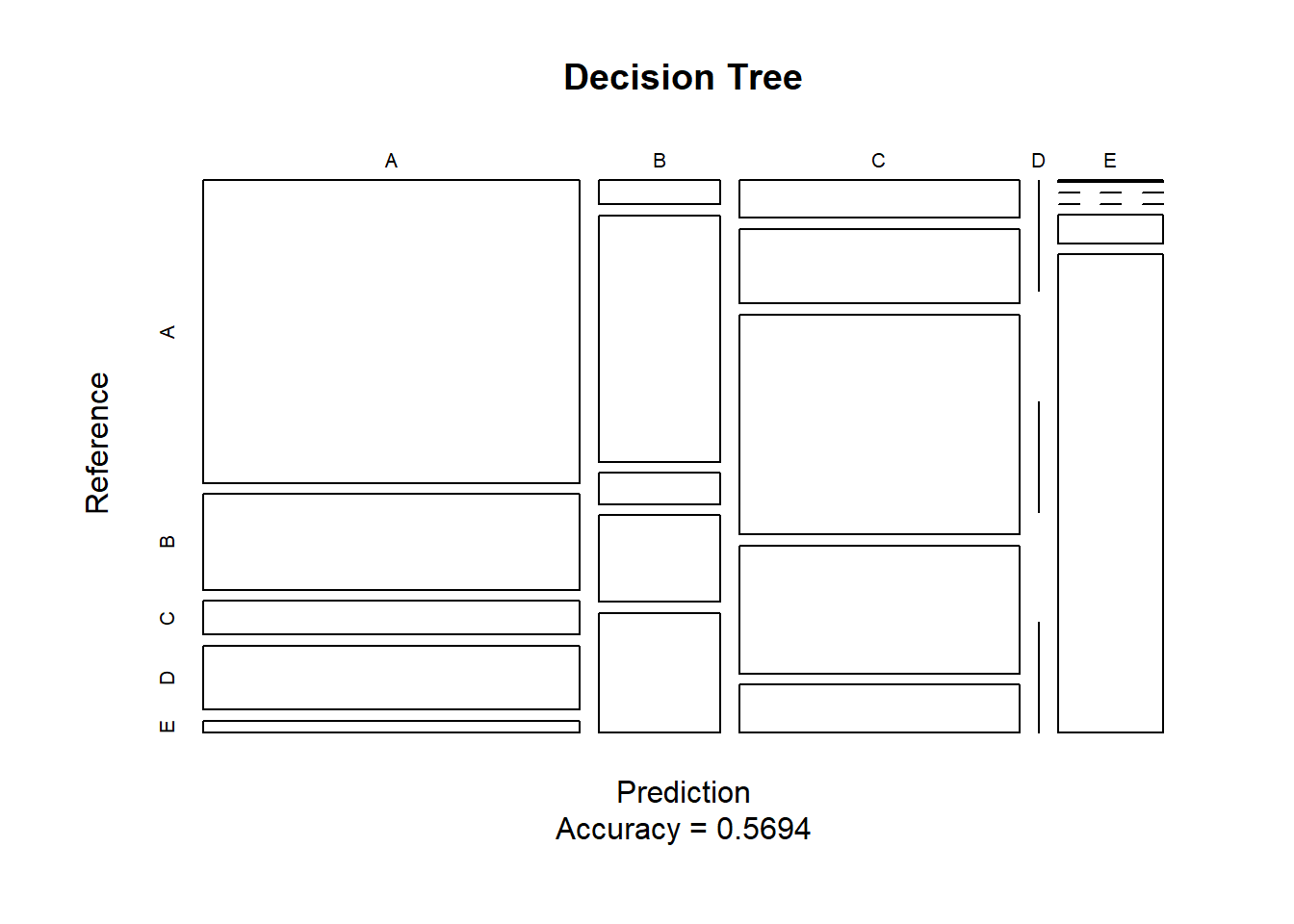### c. Generalized Boosted Model

# train model
mod.gbm = train(data = train.set,
classe ~ .,
method = "gbm",
trControl = trainControl(method = "cv",
number = 3),
verbose = F)

# test model & validate model
pred.gbm = predict(mod.gbm, newdata = test.set)

# evaluate model on validate
cm.gbm = confusionMatrix(table(pred.gbm, test.set$classe)) # plot matrix result plot(cm.gbm$table, col = cm.gbm$byClass, main = "Generalized Boosted Model", sub = paste("Accuracy =", round(cm.gbm$overall, 4)),
xlab = "Prediction",
ylab = "Reference")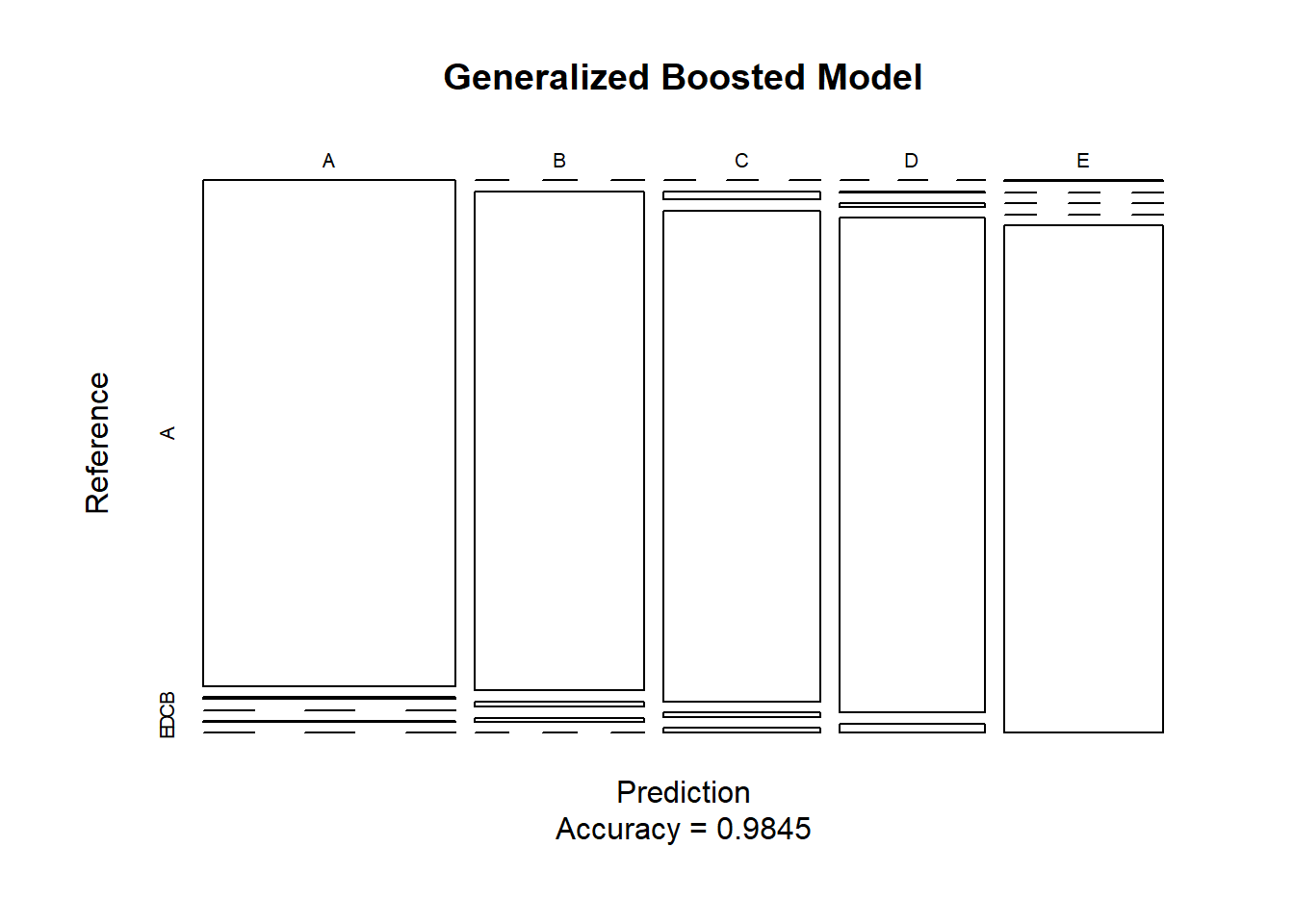## 5. Model Applying on Test Dataset

The accuracy of the 3 modeling methods above are:

• Random Forest (0.9986)
• Decision Tree (0.5694)
• Generalized Boosted Model (0.9845)
pred.test = predict(mod.rf, newdata = test)
pred.test
##   B A B A A E D B A A B C B A E E A B B B
## Levels: A B C D E

The random forest model will be applied to predict the 20 quiz results as shown above. We also used the stacking model strategy, but it was not working well with a classficiation model. Also, the accuracy was not improving.